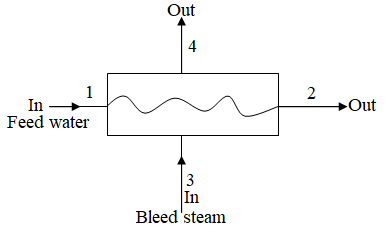The closed feedwater heater is to heat feedwater from 5000 kPa and 220 ^oC (state 1) to a...

Question:

The closed feedwater heater is to heat feedwater from {eq}5000 kPa {/eq} and {eq}220 ^oC {/eq} (state 1) to a saturated liquid (state 2). The turbine supplies bleed steam at {eq}6000 kPa {/eq} and {eq}320^oC {/eq} (state 3) to this unit. This steam is condensed to a mixture of liquid vapor (97.352% vapor and 2.648% liquid (state 4) before entering the pump. Each state indicated the position {eq}1,2,3 {/eq} and {eq}4 {/eq}. Assuming no kinetic and potential energy change, all flows assume that it is a steady state condition in control volumes, no heat loss from this closed feedwater heater (heat exchanger) to ambient, and no pressure loss in each streams ({eq}P_1=P_2 {/eq} and {eq}P_3=P_4 {/eq}).

Determine:

a) The exit temperature of feedwater ({eq}T_2 {/eq} in {eq}^oC {/eq})

b) The exit temperature of the bleed steam after passing the closed feedwater heater ({eq}T_4 {/eq}in{eq}^o C {/eq} )

c) The mass flow rate of bleed steam required to heat flowing 1 kg/s of feedwater in the unit in kg/s.

Feed Water Heater:

A component of the power plant which is used to heat pumped water from the bleed steam before entering pumped water into the boiler to reduce irreversibility of steam generation is termed as feed water heater.

Given data

• The inlet pressure of feed water heater is {eq}P_1 = 5000\;{\rm{kPa}} {/eq}
• The inlet temperature of feed water heater is {eq}T_1 = 220^\circ {\rm{C}} {/eq}
• The pressure of bleed steam is {eq}P_3 = 6000\;{\rm{kPa}} {/eq}
• The temperature of bleed steam is {eq}T_3 = 320^\circ {\rm{C}} {/eq}
• The quality of bleed steam at exit of feed water heater {eq}x = 0.97352 {/eq}
• The mass of feed water entering feed water heater is {eq}\dot m_1 = 1\;{\rm{kg/s}} {/eq}

The figure below shows the feed water heater schematics(a)

From the steam table of saturated water

The enthalpy of feed water entering the feed water heater is

{eq}h_1 = 944.383\;{\rm{kJ/kg}} {/eq}

The saturated temperature of steam at {eq}P_1 = 5000\;{\rm{kPa}} {/eq} is

{eq}T_{sat,2} = 264^\circ {\rm{C}} {/eq}

The temperature of feed water leaving the feed water heater is

{eq}T_2 = T_{sat,2} {/eq}

Substitute the value in above expression

{eq}T_2 = 264^\circ {\rm{C}} {/eq}

The enthalpy of saturated water at {eq}P_1 = 5000\;{\rm{kPa}} {/eq} is

{eq}h_{f,2} = 1154.5\;{\rm{kJ/kg}} {/eq}

The enthalpy of feed water leaving the feed water heater is

{eq}h_2 = h_{f,2} {/eq}

Substitute the value in above expression

{eq}h_2 = 1154.5\;{\rm{kJ/kg}} {/eq}

Hence exit temperature of feed water is {eq}264^\circ {\rm{C}} {/eq}.

(b)

From the steam table of super heated steam

The enthalpy of bleed steam is

{eq}h_3 = 2953.6\;{\rm{kJ/kg}} {/eq}

The saturated temperature of bleed steam is

{eq}T_{sat,4} = 275.5^\circ {\rm{C}} {/eq}

The enthalpy of saturated steam at {eq}6000\;{\rm{kPa}} {/eq} is

{eq}h_{g,4} = 2784.6\;{\rm{kJ/kg}} {/eq}

The enthalpy of saturated steam at {eq}6000\;{\rm{kPa}} {/eq} is

{eq}h_{f,4} = 1213.9\;{\rm{kJ/kg}} {/eq}

The expression for enthalpy of bleed steam is

{eq}h_4 = h_{f,4} + x\left( {h_{g,4} - h_{f,4} } \right) {/eq}

Substitute the value in above expression

{eq}\begin{align*} h_4 &= 1213.9\;{\rm{kJ/kg}} + 0.97352\left( {1229.4\;{\rm{kJ/kg}} - 1213.7\;{\rm{kJ/kg}}} \right) \\ &= 1213.9\;{\rm{kJ/kg}} + 1529.1\;{\rm{kJ/kg}} \\ &= 2743\;{\rm{kJ/kg}} \\ \end{align*} {/eq}

Hence exit temperature of bleed steam after passing the closed feed water heater is {eq}275.5^\circ {\rm{C}} {/eq}.

(c)

The expression for energy balance in feed water heater is

{eq}\dot m_1 \left( {h_2 - h_1 } \right) = \dot m\left( {h_3 - h_4 } \right) {/eq}

Here, mass flow rate of bleed steam is {eq}\dot m {/eq}.

Substitute the value in above expression

{eq}\begin{align*} 1\;{\rm{kg/s}}\left( {1154.5\;{\rm{kJ/kg}} - 944.383\;{\rm{kJ/kg}}} \right) &= \dot m\left( {2953.6\;{\rm{kJ/kg}} - 2743\;{\rm{kJ/kg}}} \right) \\ \dot m &= \dfrac{{1\;{\rm{kg/s}} \times 210.117\;{\rm{kJ/kg}}}}{{210.55}} \\ \dot m &= 0.997\;{\rm{kg/s}} \\ \end{align*} {/eq}

Hence mass flow rate of bleed steam is {eq}0.997\;{\rm{kg/s}} {/eq}.